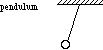index: click on a letter A B C D E F G H I J K L M N O P Q R S T U V W X Y Z A to Z index index: subject areas numbers & symbols sets, logic, proofs geometry algebra trigonometry advanced algebra & pre-calculus calculus advanced topics probability & statistics real world applications multimedia entrieswww.mathwords.com about mathwords website feedback

 Simple Harmonic Motion SHM Any kind of periodic motion that can be modeled using a sinusoid. That is, motion that can be approximately or exactly described using a sine or cosine function. Examples include the swinging back and forth of a pendulum and the bobbing up and down of a mass hanging from a spring.See also